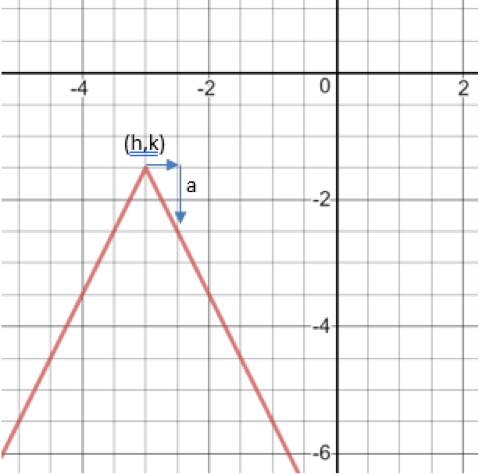# LINR 3 | Lesson 3 | Explore (Absolute Value Analysis)

Given the graph of an absolute value function, the general equation in the form $$y=a|x-h|+k$$, can be found by locating the vertex $$(h,k)$$ and dilation factor and orientation, a. Graphs are created using Desmos.com.How can we find the dilation factor from the graph? Starting at the vertex, find the rise when you run 1 to the right.

In this graph the vertex is $$(-3,-1.5)$$ and a is -2. So the equation is $$y=-2|x+3|-1.5$$.

The general function $$y=a|x-h|+k$$ can be rewritten as a piecewise function as

$y=\begin{cases} a(x-h)+k, & x-h\geq0 \\ -a(x-h)+k, & x+h<0 \end{cases}$

So, to rewrite $$y=-2|x+3|-1.5$$ as a piecewise function

$y=\begin{cases} -2(x+3)-1.5, & x+3\geq0 \\ 2(x+3)-1.5, & x+3<0 \end{cases}$

You may use the graph to confirm.

Given that information we can see find the equations for the 1st 3 graphs:

First graph:  $$a = 3$$ and $$k = 2$$;  $$y = 3|x| + 2$$

Second graph:  $$a = -3$$ and $$k = -2$$ ; $$y = -3|x| -2$$

Third graph:  $$a = 3$$ and $$h = -2$$;  $$y = 3|x+ 2|$$

Fourth graph is a piecewise function but not an absolute value function